# Sum facts for kids

Kids Encyclopedia Facts
(Redirected from Summation)

The sum of two numbers is what we get when we add the two numbers together. This operation is called summation. There are a number of ways of writing sums, with the most common being:

• Addition (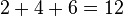$2+4+6 = 12$)
• Summation (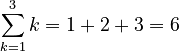$\sum_{k=1}^3 k = 1+2+3=6$)
• Computerization:
Sum = 0
For I = M to N
Sum = Sum + X(I)
Next I (in Visual BASIC)

## Sigma notation

Sigma notation is a mathematical notation to write long sums in a short way. Sigma notation uses the Greek letter Sigma, (), and takes upper and lower bounds which tell us where the sum begins and where it ends. The lower bound usually has a variable (called the index) given a value, such as "i=2". This tells us that the summation begins at 2, and goes up by 1 until it reaches the number on the top.

## Properties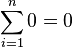$\sum_{i=1}^n 0 = 0$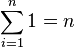$\sum_{i=1}^n 1 = n$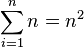$\sum_{i=1}^n n = n^2$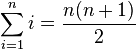$\sum_{i=1}^n i = \frac{n(n+1)}{2}$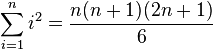$\sum_{i=1}^n i^2 = \frac{n(n+1)(2n+1)}{6}$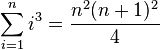$\sum_{i=1}^n i^3 = \frac{n^2 (n+1)^2}{4}$

## Applications

Sums are used to represent series and sequences. For example,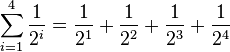$\sum_{i=1}^4 \frac{1}{2^i} = \frac{1}{2^1} + \frac{1}{2^2} + \frac{1}{2^3} + \frac{1}{2^4}$

The geometric series of a repeating decimal can be represented in summation,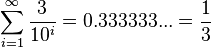$\sum_{i=1}^\infty \frac{3}{10^i} = 0.333333... = \frac{1}{3}$

The concept of an integral is a limit of sums. The area under a shape being defined as: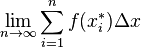$\lim_{n \to \infty} \sum_{i=1}^n f(x_i^*)\Delta x$Sum Facts for Kids. Kiddle Encyclopedia.